Next: Pair Annihilation Up: S-Matrix Previous: Electron Scattering

## Pair Creation

Next consider pair production. In this case,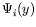atreduces to a plane wave with negative energy. This particle state propagating backward in time then represents a positron. We use the notation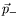,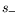for the three-momentum and spin corresponding to the physical electron and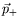,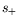for the physical positron, where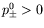. The physical positron state at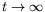is described by a plane wave of negative energy with quantum numbers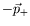,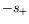,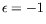. This wave propagating backward in time enters into the vertex.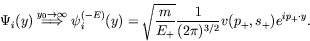(6.97)

This form of the wave function explicitly exhibits the negative energy and negative three-momentum of the wave function. The fact that the spin direction is reversed, i.e., is taken into account by the definition of the spinor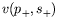. Recall that the spinors have been defined according to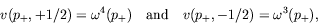(6.98)

where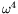is the spinor corresponding to a negative-energy electron with spin up and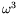a negative-energy electron with spin down.

The final wave function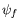in the case of pair production is a positive-energy solution carrying the quantum numbers,,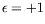and describes an electron (figure 6.9).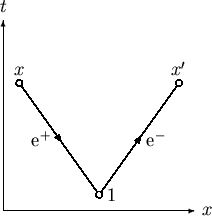The two second-order amplitudes for pair creation are shown in figure 6.10. These two second-order diagrams are said to differ in the time ordering of the two scattering processes. Since the Feynman propagator consists of two parts there is no need to deal explicity with time orderings when calculating any process. The formula for the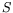-matrix automatically contains them all. However, we do not work with the general-matrix, but visualize it one term at a time, and hence one diagram at a time.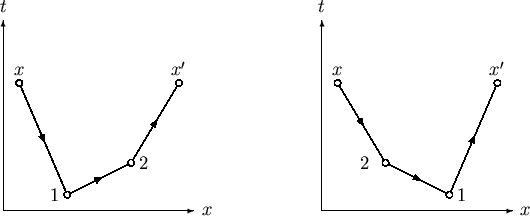Next: Pair Annihilation Up: S-Matrix Previous: Electron Scattering
Douglas M. Gingrich (gingrich@ ualberta.ca)
2004-03-18